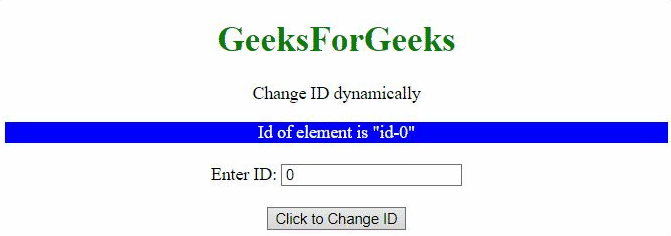GeeksforGeeks App
Open AppBrowser
Continue

# How to set id attribute of an element dynamically using AngularJS ?

In this article, we will see how to change the ID of any element dynamically using AngularJS, along with understanding its implementation through examples.

Approach 1: In this approach, a function is called when the button is clicked, which changes the ID of the element to id-5. We are calling a function on the scope variable and changing the ID from id-1 to id-5.

Example 1: This example describes setting the element’s id attribute dynamically in AngularJS.

## HTML

 ` ``<``html``>  `` ` `<``head``>``    ``<``script` `src``=``"https://ajax.googleapis.com/ajax/libs/angularjs/1.2.13/angular.min.js"``>``    ````     ` `    ``<``script``>``        ``var myApp = angular.module("app", []);``            ``myApp.controller("controller", ``            ``function(\$scope) {``            ``\$scope.id = 1;``            ``\$scope.IdChange = function() {``                ``\$scope.id = 5;``            ``};``        ``});``    `` `` ` `    ``<``style``>``        ``#id-1{ ``            ``color: red;``        ``}``        ``#id-5{``            ``color: green;``        ``}``    ````   `` ` `<``body` `style``=``"text-align:center;"``>``    ``<``h1` `style``=``"color:green;"``>  ``        ``GeeksForGeeks  ``    ``    ``    ``<``p``>``        ``Change ID dynamically``    ````    ``<``div` `ng-app``=``"app"``>  ``        ``<``div` `ng-controller``=``"controller"``>  ``            ``<``div` `id``=``"id-{{id}}"``>``                ``Id of element is "id-{{id}}" ``            ````            ``<``br``>``            ``<``button` `ng-click``=``"IdChange()"``>``                ``Click to Change ID``            ``  ``        ``  ``    `` ``   ```

Output:Approach 2: In this approach, a function is called when the button is clicked, which changes the ID from one to another. We are calling a function on the scope variable and changing the ID from id-0 to id-1, id-1 to id-2, and id-2 to id-0.

Example 2: In this example, the element’s id attribute is utilized to change the styling property.

## HTML

 ` ``<``html``>  `` ` `<``head``>``    ``<``script` `src``=``"https://ajax.googleapis.com/ajax/libs/angularjs/1.2.13/angular.min.js"``>``    ````     ` `    ``<``script``>``        ``var myApp = angular.module("app", []);``            ``myApp.controller("controller", function(\$scope) {``            ``\$scope.id = 1;``            ``\$scope.IdChange = function() {``                 ``\$scope.id = (\$scope.id + 1)%3;``            ``};``        ``});``    `` ``    ``<``style``>``        ``#id-0 { ``          ``color: white;``          ``background: blue;``        ``}``        ``#id-1 {``          ``color: white;``          ``background: green;``        ``}``        ``#id-2 {``          ``color: white;``          ``background: red;``        ``}``    ````   `` ` `<``body` `style``=``"text-align:center;"``>``    ``<``h1` `style``=``"color:green;"``>  ``        ``GeeksForGeeks  ``    `` ``    ``<``p``>``    ``Change ID dynamically``    ````    ``<``div` `ng-app``=``"app"``>  ``        ``<``div` `ng-controller``=``"controller"``>  ``            ``<``div` `id``=``"id-{{id}}"``>``                ``Id of element is "id-{{id}}" ``            ````            ``<``br``>``            ``<``button` `ng-click``=``"IdChange()"``>``                ``Click to Change ID``            ``  ``        ``  ``    `` ``   ```

Output:Approach 3: In this approach, the ID of the element is set by an <input> element.

Example 3: In this example, the <input> element is used to enter the id value that will be changed dynamically by clicking the button.

## HTML

 ` ``<``html``>  `` ` `<``head``>``    ``<``script` `src``=``"https://ajax.googleapis.com/ajax/libs/angularjs/1.2.13/angular.min.js"``>``    ```` ` `    ``<``script``>``        ``var myApp = angular.module("app", []);``            ``myApp.controller("controller", function(\$scope) {``            ``\$scope.id = 1;``            ``\$scope.IdChange = function() {``                 ``\$scope.id = \$scope.textval;``            ``};``        ``});``    `` ``    ``<``style``>``        ``#id-0 { ``          ``color: white;``          ``background: blue;``        ``}``        ``#id-1 {``          ``color: white;``          ``background: green;``        ``}``        ``#id-2 {``          ``color: white;``          ``background: red;``        ``}``    ````   `` ` `<``body` `style``=``"text-align:center;"``>``    ``<``h1` `style``=``"color:green;"``>  ``        ``GeeksForGeeks  ``    `` ``    ``<``p``>``        ``Change ID dynamically``    ````    ``<``div` `ng-app``=``"app"``>  ``        ``<``div` `ng-controller``=``"controller"``>  ``            ``<``div` `id``=``"id-{{id}}"``>``                ``Id of element is "id-{{id}}" ``            ````            ``<``br``>``            ``Enter ID: <``input` `type``=``"text"` `ng-model``=``"textval"` `>``            ``<``br``><``br``>``            ``<``button` `ng-click``=``"IdChange()"``>``                ``Click to Change ID``            ``  ``        ``  ``    `` ``   ```

Output:My Personal Notes arrow_drop_up
Related Tutorials